Next: Implementation of the code Up: Simple stellar models and Previous: Simple stellar models and   Contents

## The TOV equations

Static, spherically symmetric perfect fluid models in general relativity, and thus models of stars, are described by the Tolman-Oppenheimer-Volkoff equation.

The equations for a TOV star [4,5] are usually given in Schwarzschild coordinates. For a textbook discussion see Chapter (6.2) in the book by Wald . The notation for the fluid quantities follows . Here we are assuming a perfect fluid matter model, i.e. that the stress energy tensor is given by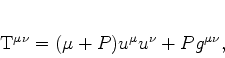(22)

where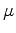is the total energy,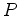the pressure and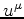is the fluid four velocity. Regarding equations of state, we will focus on the simple family of polytropic equations of state. Polytropes are self-gravitating gaseous spheres that are very usefull as a crude approximation of more realistic stellar models in astrophysics and one can go far using it. More realistic models incorporate diverse equations of state, for nuclear matter for example, or quark-gluon plasma state, etc.(23)

Where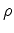is the rest-mass density. for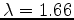corresponds to an adiabatic star supported by pressure of non-relativistic gas, and the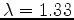case corresponds to an adiabatic star supported by pressure of ultra-relativistic gas.

The metric in Schwarzschild coordinates is given by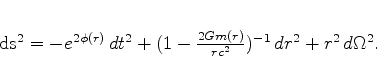(24)

Here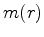is the gravitational mass inside the sphere of radius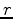, and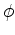is the logarithm of the lapse and can also be interpreted as the Newtonian gravitational potential.

In the exterior vacuum region we have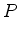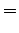(25)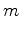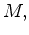(26)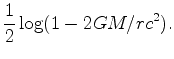(27)

This allows us to matchat the surface of the star. Note that for numerical hydrodynamical evolutions, the pressure is often set to a small nonzero value ('atmosphere') in the exterior for technical reasons.

The Einstein equations then imply the TOV system of ODEs (for a derivation see e.g.  or go ahead and try yourself whenever you have time):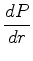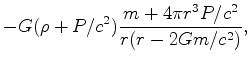(28)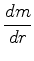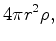(29)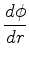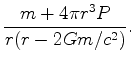(30)

Note that the last equation decouples from the first two! The initial data at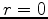is (for the numerical integration)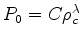,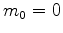,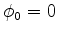. Note that the equation is singular at, this may require some attention in the numerical code!

We will choose geometric units where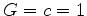4. Rewriting our metric and our equations in geometric units we have,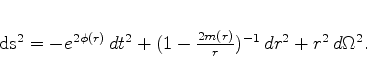(31)acts as a relativistic analog of the newtonian gravitational potential. So the equations we are going to use to describe the hydrostatic equilibrium inside our compact object is,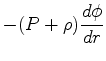(32)(33)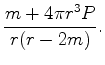(34)

where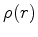and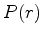are the total energy density and pressure of the fluid star, respectively. These quantities are measured in the rest frame.

An equivalent form of the TOV equations is obtained substituting (35) into (34),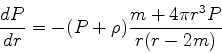(35)

as we saw before.Next: Implementation of the code Up: Simple stellar models and Previous: Simple stellar models and   Contents
Benjamin Gutierrez 2005-07-23